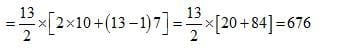# Test: Arun Sharma Based Level 1: Remainder & Divisibility

## 10 Questions MCQ Test Quantitative Aptitude (Quant) | Test: Arun Sharma Based Level 1: Remainder & Divisibility

Description
Attempt Test: Arun Sharma Based Level 1: Remainder & Divisibility | 10 questions in 15 minutes | Mock test for CAT preparation | Free important questions MCQ to study Quantitative Aptitude (Quant) for CAT Exam | Download free PDF with solutions
QUESTION: 1

### Let n! = 1 x 2 x 3 x……….x n for integer n ≥ 1. If p = 1! + (2 x 2!) + (3 x 3!) + ……(10 x 10!), then p + 2 when divided by 11! Leaves remainder of

Solution:

If P = 1! = 1
Then P + 2 = 3, when divided by 2! remainder will be 1.
If P = 1! + 2 × 2! = 5
Then, P + 2 = 7 when divided by 3! remainder is still 1.
Hence, P = 1! + (2 × 2!) + (3 × 3!)+ ……+ (10 × 10!)
Hence, when p + 2 is divided by 11!, the remainder is 1.
Alternative method:
P = 1 + 2 × 2! + 3 × 3! + …..10 × 10!
= (2 – 1)1! + (3 – 1)2! + (4 – 1)3! + …+ (11 – 1)10!
= 2! – 1! + 3! – 2! + …..+ 11! – 10!
= 11! – 1
Hence p + 2 = 11! + 1
Hence, when p + 2 is divided by 11!, the remainder is 1

QUESTION: 2

### The remainder, when (1523 + 2323) is divided by 19, is:

Solution:

a+bn is always divisible by a + when n is odd.
Therefore 1523 + 2323 is always divisible by 15 + 23 = 38.
As 38 is a multiple of 19, 1523 + 2323 is divisible by 19.
Therefore,the required remainder is 0.

QUESTION: 3

### What is the sum of all two-digit numbers that give a remainder of 3 when they are divided by 7?

Solution:

First of all, we have to identify such 2 digit numbers.
Obviously, they are 10, 17, 24, ….94
The required sum = 10 + 17 … 94.
Now this is an A.P. with a = 10, n = 13 and d = 7
Hence, the sum isQUESTION: 4

After the division of a number successively by 3, 4 and 7, the remainders obtained are 2,1 and 4 respectively. What will be the remainder if 84 divides the same number?

Solution:

In the successive division, the quotient of first division becomes the dividend of the second division and so on.
Let the last quotient be p, so the last dividend will be 7p + 4 which is the quotient of the second division.
So, the second dividend is (7p + 4) × 4 + 1.
Applying the same logic, the number = 3 {4(7p + 4) + 1} + 2 = 84p + 53
Hence, if the number is divided by 84, the remainder is 53.

QUESTION: 5

Let N = 553 + 173 – 723. N is divisible by:

Solution:

We have N = 553 + 173 – 723 = (54 + 1)3 + (18 – 1)3 – 723
When N is divided by 3, we get remainders (1)3 + (- 1)3 – 0 = 0
Hence, the number N is divisible by 3.
Again N = (51 + 4)3 + 173 – (68 + 4)3
When N is divided by 17, the remainder is (4)3 + 0 – (4)3 = 0
Hence, the number is divisible by 17.
Hence, the number is divisible by both 3 and 17.

QUESTION: 6

What will be the unit digit of 1341 ?

Solution:

As we know the last digit depends upon the unit digit of the multiplier numbers so the unit digit of 1341
is same as the last digit of 341 and we know that the cyclicity of 3 is 4
On dividing the number 41 with 4 and we will get the remainder as 1 and the last digit will be 31 = 3

QUESTION: 7

What will be the unit digit when 4545

Solution:

Here the last digit is depend upon the 5
The cyclicity of 5 is 1 so there is no need to divide the power with 1
because whatever is the power of 5 the last digit will remains 5 so the last
digit of 4545 will be 5 .
51 = 5
52 = 25
5= 125

QUESTION: 8

What will be the last digit of 3x 45 x 56

Solution:

Last digit of 3= 1
Last digit of 45 = 4
Last digit of 5= 5
So as we discussed earlier that the last digit depends upon the last digits
So 1 x 4 x 5 = 20 so the last digit is 0; also whenever 5 is multiplied by even
number then last digit will be 0

QUESTION: 9

Identify the last digit of (79+ 875)

Solution:

Last digit of 794 is depends upon the the 94 i.e 1
Last digit of 875 is depends upon the the 75i.e 7
So the last digit of the whole expression will be 1 +7 = 8
So the last digit will be 8

QUESTION: 10

What will be the last digit of 4356 x 567 x 4534

Solution:

Last digit of 4356 = 1 because cyclicity of 3 is 4 and 56 is completely
divisible by 4 so the last term of 3is 1.
Last digit of 567 is 5 and last digit of 4534 is also 5
so that means the last digit of whole of the expression is 1 x 5 x 5 = 5 therefore the digit is 5.Use Code STAYHOME200 and get INR 200 additional OFF Use Coupon Code

### How to Prepare for CAT

Read our guide to prepare for CAT which is created by Toppers & the best Teachers